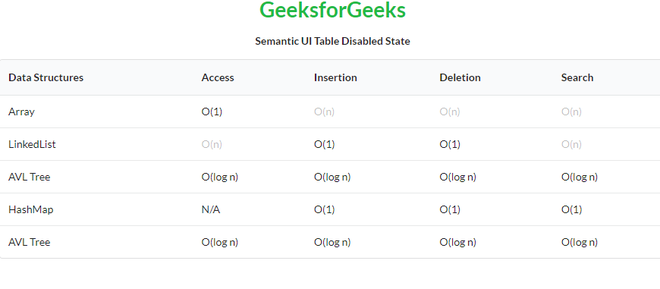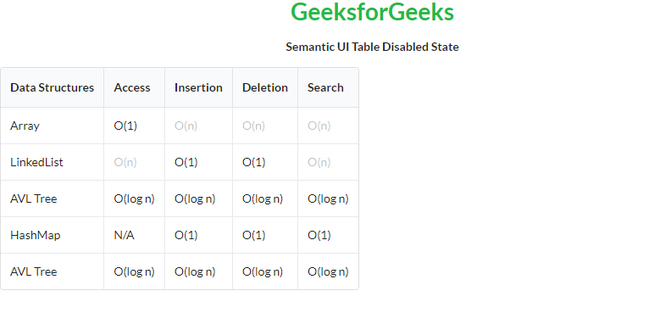GeeksforGeeks App
Open AppBrowser
Continue

# Semantic-UI Table Disabled State

Semantic UI is an open-source framework that uses CSS and jQuery to build great user interfaces. It is the same as a bootstrap for use and has great different elements to use to make your website look more amazing. It uses a class to add CSS to the elements. Tables are an easy way to organize a lot of data. A table is an arrangement of data in rows and columns, or possibly in a more complex structure. Tables are widely used in communication, research, and data analysis. Tables are useful for various tasks such as presenting text information and numerical data. It can be used to compare two or more items in the tabular form layout. Tables are used to create databases. An HTML table and a Semantic UI table both are the same structurally.

A disabled State can disable a cell.

Semantic UI Table Disabled State Classes:

• table: This class creates a table in Semantic UI.
• disabled: This class disables the selected cell.
• celled: This class divides each row of our table into separate cells.

Syntax:

```<table class="ui table">
<tr>
<td class="disabled"></td>
...
</tr>
...
</table>```

Example 1: This is a basic example illustrating Table Disabled State made using Semantic UI.

## HTML

 ```<``html``>`` ` `<``head``>``    ``<``title``>Semantic UI Table Disabled State``    ``<``link` `href``=``"https://cdnjs.cloudflare.com/ajax/libs/semantic-ui/2.4.1/semantic.min.css"` `    ``rel``=``"stylesheet"` `/>``    ``<``script` `src``=``    ``"https://code.jquery.com/jquery-3.1.1.min.js"``        ``integrity``=``        ``"sha256-hVVnYaiADRTO2PzUGmuLJr8BLUSjGIZsDYGmIJLv2b8="` `        ``crossorigin``=``"anonymous"``> ``    ````    ``<``script` `src``=``"https://cdnjs.cloudflare.com/ajax/libs/semantic-ui/2.4.1/semantic.min.js"``>``    `````` ` `<``body``>``    ``<``center``>``        ``<``h1` `class``=``"ui green header"``>GeeksforGeeks``        ``<``strong``>Semantic UI Table Disabled State``    ````    ``<``table` `class``=``"ui table"``>``        ``<``thead``>``            ``<``tr``>``                ``<``th``>Data Structures``                ``<``th``>Access``                ``<``th``>Insertion``                ``<``th``>Deletion``                ``<``th``>Search``            ````        ````        ``<``tbody``>``            ``<``tr``>``                ``<``td``>Array``                ``<``td``>O(1)``                ``<``td` `class``=``"disabled"``>O(n)``                ``<``td` `class``=``"disabled"``>O(n)``                ``<``td` `class``=``"disabled"``>O(n)``            ````            ``<``tr``>``                ``<``td``>LinkedList``                ``<``td` `class``=``"disabled"``>O(n)``                ``<``td``>O(1)``                ``<``td``>O(1)``                ``<``td` `class``=``"disabled"``>O(n)``            ````            ``<``tr``>``                ``<``td``>AVL Tree``                ``<``td``>O(log n)``                ``<``td``>O(log n)``                ``<``td``>O(log n)``                ``<``td``>O(log n)``            ````            ``<``tr``>``                ``<``td``>HashMap``                ``<``td``>N/A``                ``<``td``>O(1)``                ``<``td``>O(1)``                ``<``td``>O(1)``            ````            ``<``tr``>``                ``<``td``>AVL Tree``                ``<``td``>O(log n)``                ``<``td``>O(log n)``                ``<``td``>O(log n)``                ``<``td``>O(log n)``            ````        ````    `````` ` ``

Output:Example 2: This is a basic example illustrating Celled and Collapsing Table Disabled State made using Semantic UI.

## HTML

 ```<``html``>`` ` `<``head``>``    ``<``title``>Semantic UI Table Disabled State``    ``<``link` `href``=``"https://cdnjs.cloudflare.com/ajax/libs/semantic-ui/2.4.1/semantic.min.css"` `    ``rel``=``"stylesheet"` `/>``    ``<``script` `src``=``"https://code.jquery.com/jquery-3.1.1.min.js"``        ``integrity``=``"sha256-hVVnYaiADRTO2PzUGmuLJr8BLUSjGIZsDYGmIJLv2b8="` `        ``crossorigin``=``"anonymous"``> ``    ````    ``<``script` `src``=``"https://cdnjs.cloudflare.com/ajax/libs/semantic-ui/2.4.1/semantic.min.js"``>`````` ` `<``body``>``    ``<``center``>``        ``<``h1` `class``=``"ui green header"``>GeeksforGeeks``        ``<``strong``>Semantic UI Table Disabled State``    ````    ``<``table` `class``=``"ui table celled collapsing"``>``        ``<``thead``>``            ``<``tr``>``                ``<``th``>Data Structures``                ``<``th``>Access``                ``<``th``>Insertion``                ``<``th``>Deletion``                ``<``th``>Search``            ````        ````        ``<``tbody``>``            ``<``tr``>``                ``<``td``>Array``                ``<``td``>O(1)``                ``<``td` `class``=``"disabled"``>O(n)``                ``<``td` `class``=``"disabled"``>O(n)``                ``<``td` `class``=``"disabled"``>O(n)``            ````            ``<``tr``>``                ``<``td``>LinkedList``                ``<``td` `class``=``"disabled"``>O(n)``                ``<``td``>O(1)``                ``<``td``>O(1)``                ``<``td` `class``=``"disabled"``>O(n)``            ````            ``<``tr``>``                ``<``td``>AVL Tree``                ``<``td``>O(log n)``                ``<``td``>O(log n)``                ``<``td``>O(log n)``                ``<``td``>O(log n)``            ````            ``<``tr``>``                ``<``td``>HashMap``                ``<``td``>N/A``                ``<``td``>O(1)``                ``<``td``>O(1)``                ``<``td``>O(1)``            ````            ``<``tr``>``                ``<``td``>AVL Tree``                ``<``td``>O(log n)``                ``<``td``>O(log n)``                ``<``td``>O(log n)``                ``<``td``>O(log n)``            ````        ````    `````` ` ``

Output:My Personal Notes arrow_drop_up
Related Tutorials